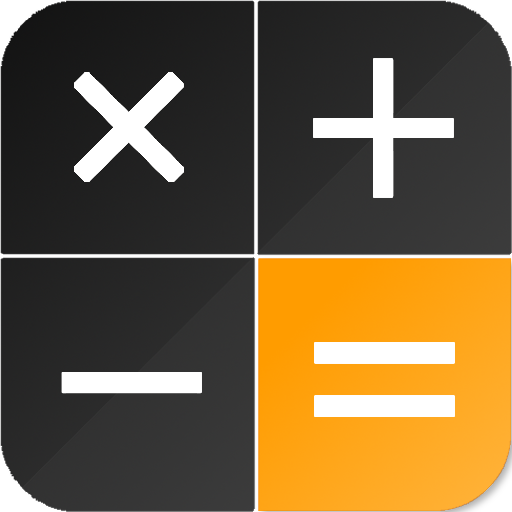# Voting Now

##### Creativity
×## CalQwik Calculator

### by Tricky Maths

Free
Design
Usability
Creativity
Average
13 USERS VOTED
##### TAGS
###### Description

CalQwik is a free android calculator and unit converter app that will let you replace your built-in calculator. It is easy to use and perfect for everyday calculations.

It has many features, like:

1) Calculator with four basic arithmetic functions (addition, subtraction, division, multiplication), parentheses, percent function, memory functions, trigonometric functions, exponents, squares, cubes, and square roots and cube roots.

2) Unit Converter supporting units for length, weight, area, time, speed, pressure, energy, power, data storage and volume. Get units converted as you type.

3) Choose from 26 stylish and clean themes.

4) Calculates quickly as you type. Copy result by just tapping the result. Share current
equation with result by tapping on share button on top of screen.ca

5) Possible to modify equation as you like. No need to delete full equation and re-typing it. You can also paste equations and get results.

6) Access quick history with date and time by swiping keypad to right. Each equation in history has its dedicated share, copy and delete function.

7) Extended history is available where you can copy, share and delete more than one equation.

8) You can tap on any equation in quick history to replace current equation and result by those in history.

9) Long press equation or result in quick history to insert it in current equation.

10) It shows results and equation with group separations.

View All